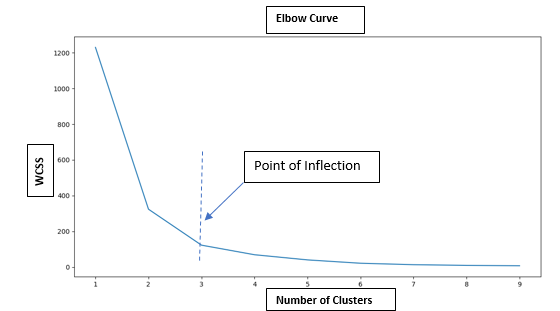....

Clustering

Machine Learning

Unsupervised Learning

January 15, 2021

# Discuss the elbow method.

In K-Means algorithm we need to specify the number of clusters. As is the inherent nature of the algorithm, with increase in number of clusters the WCSS decreases. But the rate of decrease is steep for first few points and then plateaus. Thus the curve resembles the shape of human elbow, hence the name. The point of inflection is the ideal number of cluster for the given dataset.by : Monis Khan

Quick Summary:

In K-Means algorithm we need to specify the number of clusters. As is the inherent nature of the algorithm, with increase in number of clusters the WCSS decreases. But the rate of decrease is steep for first few points and then plateaus. Thus the curve resembles the shape of human elbow, hence the name. The […]